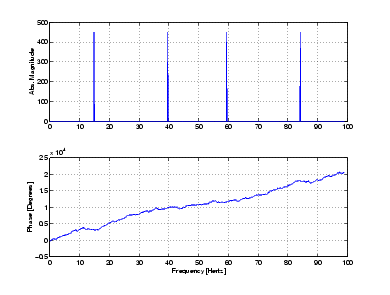MathematicsMagnitude and Phase of Transformed Data

Important information about a transformed sequence includes its magnitude and phase. The MATLAB functions `abs` and `angle` calculate this information.

To try this, create a time vector `t`, and use this vector to create a sequence `x` consisting of two sinusoids at different frequencies.

• ```t = 0:1/100:10-1/100;
x = sin(2`*`pi`*`15`*`t) + sin(2`*`pi`*`40`*`t);
```

Now use the `fft` function to compute the DFT of the sequence. The code below calculates the magnitude and phase of the transformed sequence. It uses the `abs` function to obtain the magnitude of the data, the `angle` function to obtain the phase information, and `unwrap` to remove phase jumps greater than `pi` to their `2*pi` complement.

• ```y = fft(x);
m = abs(y);
p = unwrap(angle(y));
```

Now create a frequency vector for the x-axis and plot the magnitude and phase.

• ```f = (0:length(y)-1)'*100/length(y);
subplot(2,1,1), plot(f,m),
ylabel('Abs. Magnitude'), grid on
subplot(2,1,2), plot(f,p*180/pi)
ylabel('Phase [Degrees]'), grid on
xlabel('Frequency [Hertz]')
```

The magnitude plot is perfectly symmetrical about the Nyquist frequency of 50 hertz. The useful information in the signal is found in the range 0 to 50 hertz.Introduction FFT Length Versus Speed